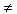Introduction

The standard form of a quadratic equation is ax2 + bx + c = 0 where a, b, and c are real numbers and a0. Earlier in this unit, vertex form and factored form were discussed. There is a similarity between all three forms. The "a" in the standard form is the same "a" as in the factored and vertex form. That is, the a will always have exactly the same value. To determine the values of a, b, and c, write the equation in standard form.

Example 1 Find the a, b, and c values of the equation: x2 - 3x = 28.

Step 1. Put the equation in standard form.

1x2 - 3x - 28 = 0

Step 2. Identify the a, b, and c values.

a = 1, b = -3, c = -28

Example 2 Find the a, b, and c values of the equation: 5x2 = -45.

Step 1. Put the equation in standard form.

5x 2 + 0x + 45 = 0

Step 2. Identify the a, b, and c values.

a = 5, b = 0, c = 45